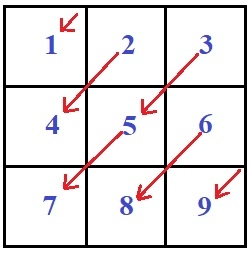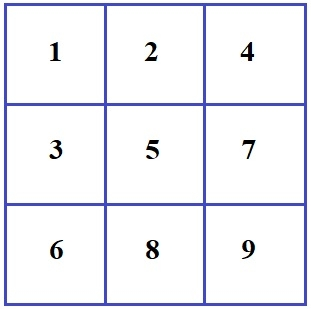# Print numbers in matrix diagonal pattern in C Program.

The task is to print the matrix of n x n of the diagonal pattern.

If n is 3 then to print a matrix in Diagonal pattern is −So the output will be like −## Example

Input: 3
Output:
1 2 4
3 5 7
6 8 9
Input: 4
Output:
1 2 4  7
3 5 8 11
6 9 12 14
10 13 15 16

The problem suggests we have to give a number n and generate a matrix of n x n and then we have to traverse the matrix in a diagonal pattern and store the values in a separate matrix.

But this will increase the complexity of our code, so we will −

• Create a matrix of size N X N which will store the pattern before printing.

• Store the elements in the upper triangle of the pattern. As observed the row index increases by 1 and the column index decreases by 1 as you move down the diagonal.

• Once the upper triangle is completed then store the elements of the lower triangle in a similar way as the upper triangle i.e. row index increases by 1 and column index decreases by 1 as you move down the diagonal.

## Algorithm

int printdiagonal(int n)
START
STEP 1: DECLARE int mat[n][n], i, j, k, d=1, m
STEP 2: LOOP FOR i = 0 AND i < n AND i++
ASSIGN j AS i AND k AS 0
LOOP FOR j = I AND j >= 0 AND j--
ASSIGN mat[k][j] AS d
INCREMENT d AND k BY 1
END LOOP
END LOOP
STEP 3: LOOP FOR k = 1 AND k < n AND k++
ASSIGN i AND m EQUALS TO k
LOOP FOR j = n-1 AND j >= m AND j--
ASSIGN mat[i][j] AS d;
INCREMENT d AND i WITH 1
END FOR
END FOR
STEP 4: LOOP FOR i = 0 AND i < n AND i++
LOOP FOR j = 0 AND j < n AND j++
PRINT mat[i][j]
END FOR
PRINT NEWLINE
END FOR
STOP

## Example

#include <stdio.h>
int printdiagonal(int n){
int mat[n][n], i, j, k, d=1, m;
for ( i = 0; i < n; i++){
j = i;
k = 0;
for ( j = i; j >= 0; j--){
mat[k][j] = d;
d++;
k++;
}
}
for ( k = 1; k < n; k++){
i = m = k;
for ( j = n-1; j >= m; j--){
mat[i][j] = d;
d++;
i++;
}
}
for ( i = 0; i < n; i++){
for(j = 0; j < n; j++){
printf("%d ", mat[i][j] );
}
printf("");
}
}
int main(int argc, char const *argv[]){
int n = 3;
printdiagonal(n);
return 0;
}

## Output

If we run the above program then it will generate the following output −

1 2 4
3 5 7
6 8 9